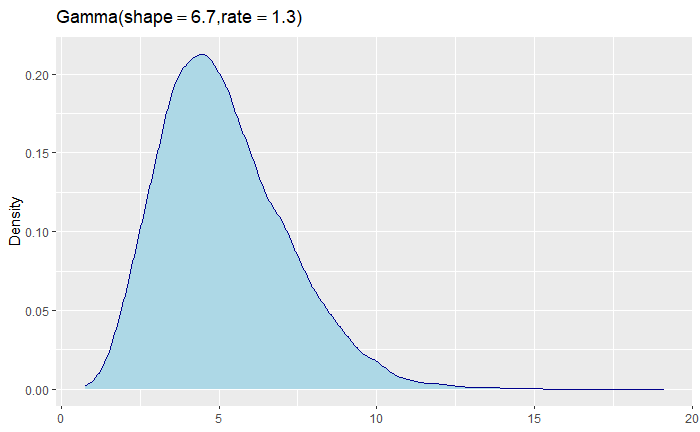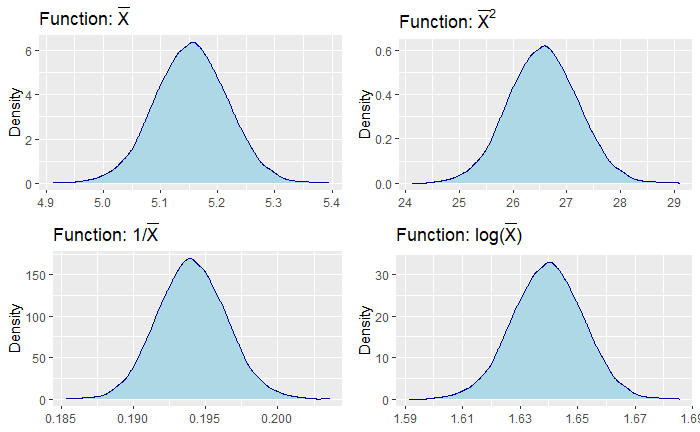# Intuition of the Delta Method in Statistics

Delta method is a useful technique when we are interested in the asymptotic probability distribution of a function of random variables. In statistics, we often come across complicated functions for which it is difficult to calculate the exact distribution. Delta Method tells us that if we have a large enough sample, then the function will follow normal distribution, if a certain condition is met.

# An Example

Let us consider that we have a sample of size n where the observations are coming from a normal distribution with mean 2 and variance 5. We can easily calculate the exact distribution of the sample mean. But what if we need to find out the distribution of a very complicated function of the sample mean. E.g., exp(exp(sample_mean)). Calculating an exact distribution could be hard. This is the kind of situations where Delta method can help us obtain an approximate distribution.

# The Delta Method

If Xₙ is a function (in formal setting, it is referred to as a sequence of X), and if Xₙ follows normal distribution asymptotically (meaning the sample size n is very large), which means,

then any function of Xₙ, which I will refer to as target function g(⋅), will follow normal distribution. Formally,

So going back to our earlier example, if sample mean

follows normal distribution then any function of the sample mean will follow normal distribution as well.

# Demonstration

For the demonstration, I will draw 10,000 observations from gamma distribution, Xᵢ ∼ Γ(α= 6.7, β = 1.3). From the plot below, we can see that the distribution is not symmetric as it is skewed towards one side.Gamma distribution with parameters shape = 6.7 and rate = 1.3

Now for us to use the delta method, we will need to find a function of Xᵢ that follows normal distribution asymptotically. This is where we can take help from the central limit theorem (CLT). CLT is a famous theorem in statistics, and there are variations of it, but a common form is expressed as,

So, CLT in this context tells us that as long as X₁, X₂….X₁₀₀₀₀ all are coming from the same distribution (not necessarily from normal distribution), then the average of those observations will approximately follow normal distribution. Now that we know the distribution of the sample mean, we can use the delta method to obtain the distribution of any function of the sample mean.

We will look into the following three target functions for this demo. Obviously, depending on he problem, there could be more complicated functions.

Since I like computational stuff more than the mathematical stuff, I will do a simulation to see if indeed these different functions of the sample mean would follow the normal distribution (provided, sample size is large). Following is the code that I used for this demo.

# generating data B = 10,000 samples each with sample size of 10,000shape_val = 6.7 rate_val = 1.3 samplesize = 10000 B = 10000 x = matrix(NA, nrow = B, ncol = samplesize) # placeholder for storing the observations# generating dataset.seed(1)for (b in 1:B){  x[b, ] = rgamma(samplesize, shape = shape_val, rate = rate_val)}# calculating meanall_means = apply(x, 1, mean)# plot functionplot_fn = function(input){  p1 = ggplot(as.data.frame(input), aes(x=input)) +     geom_density(color="darkblue", fill="lightblue") +     labs(title=TeX(r'(Function: $\bar{X}$)'), x= NA, y = "Density")+    theme(axis.title.x = element_blank())  p2 = ggplot(as.data.frame(input^2), aes(x=input^2)) +     geom_density(color="darkblue", fill="lightblue") +     labs(title=TeX(r'(Function: $\bar{X}^2$)'), x= NA, y = "Density") +    theme(axis.title.x = element_blank())  p3 = ggplot(as.data.frame(1/input), aes(x=1/input)) +     geom_density(color="darkblue", fill="lightblue") +     labs(title=TeX(r'(Function: $1/\bar{X}$)'), x= NA, y = "Density") +    theme(axis.title.x = element_blank())  p4 = ggplot(as.data.frame(log(input)), aes(x=log(input))) +     geom_density(color="darkblue", fill="lightblue") +     labs(title=TeX(r'(Function: $log(\bar{X})$)'), x= NA, y = "Density") +    theme(axis.title.x = element_blank())    grid.arrange(p1, p2, p3, p4, nrow = 2)    }plot_fn(all_means)

I generate B = 10,000 number of samples each of size n = 10,000. Then I calculate mean for each of the samples. So we have 10,000 means. If we plot the density plot of those 10,000 means, we can see that they approximately follow the normal distribution (the plot on the upper left corner). It is expected since that is what the CLT tells us. But it is good to see an example of that phenomenon. Now, the other three plots for the three different functions of the sample mean, and we can see that they approximately follow the normal distribution as well, which is what Delta method told us.All the distributions are asymptotically normal as suggested by the delta method

# Final Thoughts

Delta method is a mathematical tool, which means that we will need to use mathematical techniques to derive the distribution of the target function g(⋅). So, my method of demonstrating the delta method with a computational approach is probably not going to be very useful when you have to apply delta method for your own problems. However, examples like these help me understand what is meant by the abstract mathematical statements, and hopefully will help some of you to get a better intuition.

--

--